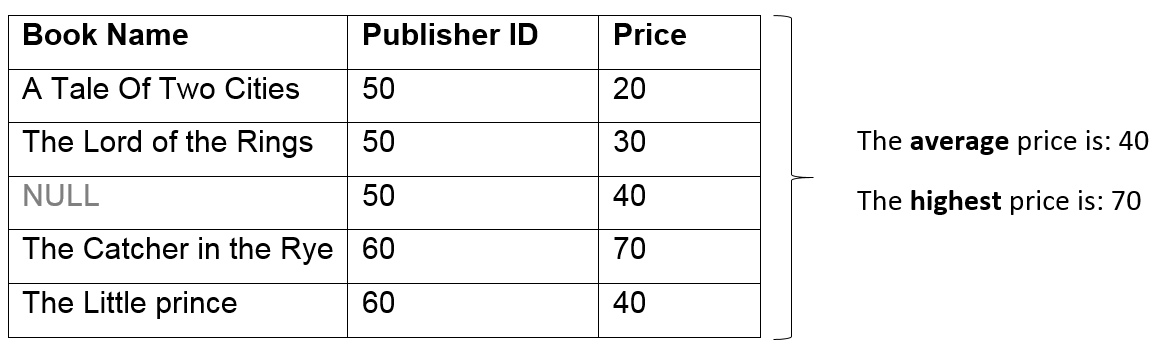Select PageThis SQL tutorial focuses on the MySQL Group Functions, and provides explanations, examples and exercises. For this lesson’s exercises use this link.

This tutorial is a part of several posts explaining how to use the MySQL Group Functions. To read additional posts regarding this subject, please use the following links:

## MySQL Group Functions

Unlike MySQL Scalar Functions, MySQL Group Functions process the values of multiple rows to give one result per group, for example :## MySQL Common Group Functions

 Function Description Syntax SUM Returns the total sum ```SELECT SUM(price) FROM books -- Result: 200 ``` MIN Returns the lowest value ```SELECT MIN (price) FROM books -- Result: 20 ``` MAX Returns the highest value ```SELECT MAX(price) FROM books -- Result: 70 ``` AVG Returns the average value ```SELECT AVG(price) FROM books -- Result: 40 ``` COUNT (*) Returns the number of records in a table ```SELECT COUNT(*) FROM books -- Result: 5 ``` COUNT (column) Returns the number of values (NULL values will not be counted) of the specified column ```SELECT COUNT(product_name) FROM books -- Result: 4 ``` COUNT (DISTINCT column) Returns the number of distinct values ```SELECT COUNT(DISTINCT publisherID) FROM books -- Result :2 ```

* Results based on the illustration mentioned above

## MySQL GROUP Functions and NULL

• In MySQL, All Group functions ignore NULL values. For example: the average price is calculated based on the rows in the table where a valid value is stored (the total price divided by the number of books with a price).
• In MySQL You can use the IFNULL function to force group functions to include NULL values, in the following example the average is calculated based on all rows in the table, regardless of whether null values are stored in the price column (the total price divided by the total number of rows in the table):
```SELECT       AVG(IFNULL(price,0))
FROM         books
```